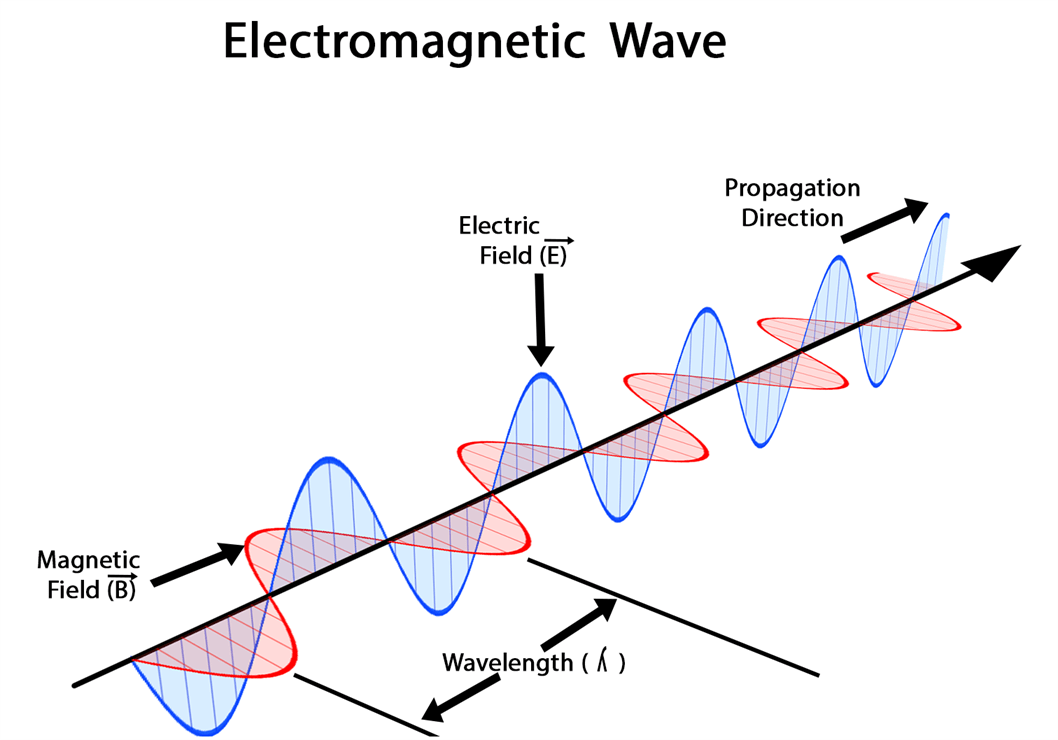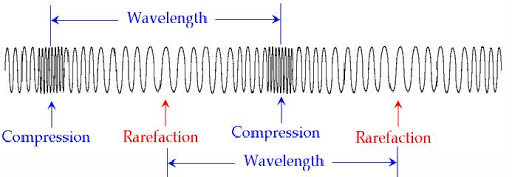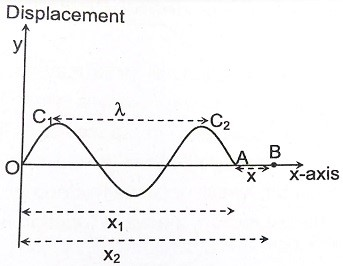Notice: Undefined index: options in /home/u337987201/domains/classnotes.ng/public_html/wp-content/plugins/elementor-pro/modules/theme-builder/widgets/site-logo.php on line 192
Wave Motion - ClassNotes.ng

# Wave Motion

Welcome to class!

In today’s class, we will be talking about wave motion. Enjoy the class!

### Wave MotionWave motion is the transfer of energy and momentum from one point of the medium to another point of the medium without actual transport of matter between two points. Wave motion is classified into three different ways they are,

1. The medium of propagation,
2. The dimensions in which a wave propagates energy
3. The energy transfer

Classification of Wave Motion: Based on the Medium of PropagationClassification of Wave Motion Based on the Medium of Propagation

Number of Dimensions a Wave Propagates Energy#### BASED ON THE TRANSFER OF ENERGY

1. Standing waves (or stationary waves)
2. Progressive wave

Standing waves remain confined to a region without any transfer of energy and momentum whereas the progressive waves transfer energy and momentum between the particles of the medium.

##### MECHANICAL WAVES (ELASTIC WAVES)

The waves which require a material medium for their propagation are known as mechanical waves. The particles of the medium execute periodic motion about a mean position when the wave propagates through the medium.

Examples of mechanical waves

1. Water waves
2. Sound waves
3. Spring waves
4. Waves of the tuning fork
###### Properties of Medium for Mechanical Wave Propagation
1. The medium must possess inertia so that its particles can store kinetic energy.
2. The medium must possess elasticity.
3. The minimum frictional force between the particles of the medium.
##### NON-MECHANICAL WAVES

Waves which do not require a medium for their propagation are called a non-mechanical wave. These types of waves can propagate through vacuum also. These are transverse in nature. For example, electromagnetic waves and matter waves.

###### Transverse Wave Motion

The particles of the medium vibrate in a direction perpendicular to the direction of propagation of the wave. The region of maximum upward displacement is called the crest region of maximum downward displacement is called the trough.

Transverse wave motion occurs only through a medium which has rigidity modulus or shape conservation. For example, string waves.##### Electromagnetic Waves

Electromagnetic waves are those that do not require a material medium for their propagation. They arise from the vibrations of Electric (E) and magnetic (m) fields. The combination between electric field and magnetic field waves is called an Electromagnetic (E-m) wave. Examples of E-m waves are lightwave, x-ray and gamma-ray etc. Electromagnetic waves are a family of the wave spectrum. The members and their frequency ranges are as follows:S/N Name Frequency Properties/Applications 1 Radio wave Use in radio communication. Examples include radar, TV waves, microwaves etc. they are generated in radio transmitters. 2 Infrared It is used in remote controls. It can be used as an alternative to visible light in photography. An infrared camera can take pictures in the dark without using flasher. 3 Visible light 107 Gives vision. 4 Ultraviolet Prevented from reaching us by the ozone layer. Dangerous to the skin. 5 X-ray 1010 Useful in taking photographs in hidden tissue, broken bones etc. 6 Gamma-ray 1011 Highly penetrating wave. It could be very dangerous to human cell and life. Useful in killing cancerous cells.
###### Difference between Mechanical and Electromagnetic waves
 Mechanical Waves Electromagnetic Waves It may be transverse or longitudinal Always transverse Travel at speed less than light Travel at the speed of light
##### LONGITUDINAL WAVE MOTION

The particles of the medium vibrate about their equilibrium position in a direction parallel to the direction of propagation of the wave is called a longitudinal waves.

Longitudinal waves require a medium with only elasticity of volume (or Bulk modulus) for its propagation. In this type of wave motion, the waves travel through a medium in the form of compression and rarefaction.The region of high pressure is called a compression and the region of low pressure is called rarefaction. For example, Sound waves in the tube.

###### Periodic Wave Motion
1. If the disturbance is continuous and is periodic in nature, then the wave produced is termed as a periodic wave.
2. A periodic wave that is varying sinusoidally is called a sinusoidal periodic wave.
3. The particles of the medium execute simple harmonic motion (SHM) when a sinusoidal periodic wave passes through the medium.
###### Characteristics of Wave Motion
1. In wave motion, the disturbance travels through the medium due to repeated periodic oscillations of the particles of the medium about their mean position (or) Equilibrium position.
2. Energy and momentum are transferred from one point to another without any actual transfer of the particles of the medium.
3. The velocity of the particle is maximum at the mean position and zeroes at the extreme position.###### Terminologies in Progressive Wave Motion
1. Amplitude
2. Period
3. Wavelength
4. Frequency
5. Wave velocity
6. Phase or phase angle (O)
7. Phase difference
8. Path difference
9. Time difference
###### Amplitude (A)

The amplitude of a wave is the maximum displacement of any particle of the medium from its equilibrium position.

###### Period (T)

Period (T) of a wave is the time taken by any particle of the medium to complete one vibration during a period (T).###### Wavelength (λ)

Wavelength (λ) is equal to the distance between two consecutive particles of the medium which are in the same state of vibration it is equal to the distance travelled by the wave by its time period (T).

###### Frequency (f)

It is the number of vibrations made per second by any particles of the medium (f = 1/T). Since the frequency of a wave is a characteristic property of the source which is producing the wave motion, hence, the frequency of a wave does not change when a wave travels from one medium to another medium.

###### Phase or Phase Angle (Φ)

It represents the state of vibration of the particle of a medium with respect to its mean position.

###### Phase Difference Δ(Φ)

It represents the different state of vibration of a particle at two different instants (or) any pair of particles at the same instant. ΔΦ = Φ2 – Φ1.

###### Wave Velocity (v)

It is the distance travelled by the wave in one second (v = λ/T). It is determined by the mechanical properties of the medium through which the wave propagates. There are two velocities associated with a wave. One is the wave velocity and the other one is particle velocity.

###### Path Difference (Δx) or (x)

It indicates the distance between two points measured along the direction of propagation of the wave through the medium.

###### Time Difference (ΔT)

It indicates the time taken by the wave to travel from one point to another through the medium.

###### The relationship between Path Difference and Phase Difference
 Units wavelength metre m displacement metre m period second s frequency Hertz (Hz) s-1 velocity metres per second ms-1

Consider a progressive wave motion advancing in the positive direction of the x-axis###### Path Difference vs Phase Difference

Let A and B be two points in the medium through which the wave passes.

The path difference between A and B is, x = x2 – x1

By the time the wave reaches B from A the phase of vibration of A has changed. The difference between the states of vibration of A and B is called phase difference (ΔO).

From this wave motion, if we consider any two consecutive crests c1 and c2, the path difference between them is λ, the time difference is T and the phase difference is 2π.

path difference of (λ) corresponds to a phase difference of 2π, thus, a path difference (x) corresponds to the phase difference 2πr/λ.

Δϕ = (2πx)/λ = 2π/λ (path difference)

Where k = 2π/λ is called wave number or propagation constant of the wave motion.

A path difference (λ) corresponds to a time difference (T), therefore, a path difference (x) corresponds to a time difference of (x/λ)T.

###### EVALUATION
1. State five Uses of Electromagnetic waves.
2. Arrange the following electromagnetic waves in the order of increasing wavelength, ultraviolet, gamma rays, radio waves, infrared, visible light, X-rays. Which has the highest frequency?

In our next class, we will be talking about Properties of Waves.  We hope you enjoyed the class.

Should you have any further question, feel free to ask in the comment section below and trust us to respond as soon as possible.

For more class notes, videos, homework help etc download our Mobile App HERE

Join ClassNotes.ng Telegram Community HERE for exclusive content and support

### 3 thoughts on “Wave Motion”

1.This is very at lesson site thanks so much

2.osazeeosaretin56

this is amazing,thanks alot

3.Wow that was so great, I love it

Don`t copy text!